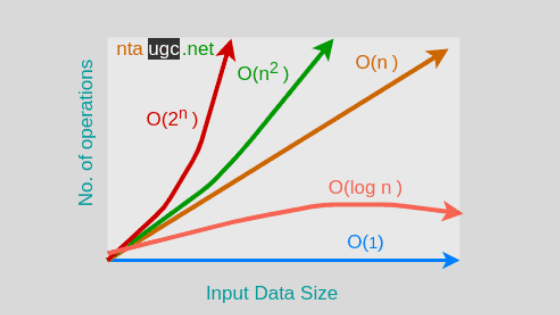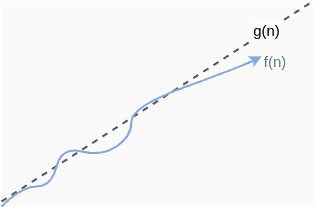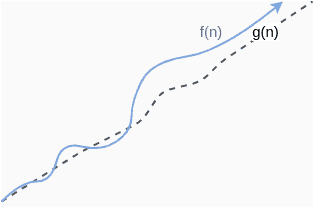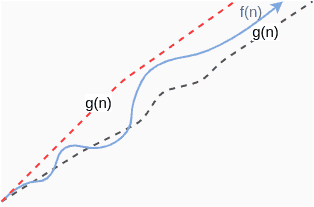# Time Complexity and Space Complexity## Time Complexity and Space Complexity

How do you do “measure” your code? Would you clock “how long” it takes to run?

What if you are running the same program on a mobile device or a quantum computer? The same code will give you different results.

To answer these questions, we need to nail some concepts first, like time complexity!

## What is time complexity, space complexity, Asymptotic analysis, asymptotic notation, big O notation?

Before reading (time complexity and space complexity) these topics in detail, we try to know one by one what the meanings of these terms actually are.

### Time Complexity

Time complexity told the time taken by the algorithm with respect to the input size

The time complexity is not about how long the algorithm takes. Instead, how many operations are executed. The number of instructions executed by a program is affected by the input’s size and how their elements are arranged.

Time complexity (or running time) is the estimated time an algorithm takes to run. However, you do not measure time complexity in seconds, but as a function of the input.

### Space Complexity

space complexity of an algorithm is overall space taken through the algorithm with respect to the input size. space complexity consists of both Auxiliary space and space utilized by input.

### Asymptotic Analysis

Asymptotic analysis is a mathematical way where we computing the running time of any operation.

Using asymptotic analysis we can very well predict an algorithm’s best case, average case, and worst-case scenario.

Usually, the time required by an algorithm falls under three types −

• Best Case − Minimum time required for program execution.

• Average Case − Average time required for program execution.

• Worst Case − Maximum time required for program execution.

1<logn<√n<n<nlogn<n²<n³<…<2^n<n^n

### Asymptotic Notation

Following are the commonly used asymptotic notations to calculate the running time complexity of an algorithm.

• Ο Notation (Big Oh Notation)
• Ω Notation (Omega Notation)
• θ Notation (Theta Notation)

## Big Oh NotationThe notation  Ο(n) is the formal way of representing the upper bound of an algorithm’s running time.

It calculates the time complexity of the worst-case scenario or the longest period of time an algorithm could take to complete.

Definition:

f(n) = O(g(n)) (f is a Big-O of g) or f<= g if there exist constants N and c so that for all n=>N, f(n) <= c.g(n)

Big O really just says that my runtime is sort of bounded above by some multiple of this thing. sometimes you want to say the reverse. sometimes you want to say that I am bounded below. And so there is the different notation for that Omega Notation and Theta Notation respectively.

## Omega NotationThe notation  Ω(n) is the formal way of representing the lower bound of an algorithm’s running time.

It calculates the time complexity of the best-case scenario or the best amount and the smallest period of time an algorithm could take to complete.

Defination:

For functions f,g : N -> R+ we say that:

f(n) =  Ω(g(n)) or f>=g if for some c, f(n) >= c.g(n)  {f grows no slower than g}

## Theta NotationThe notation θ (n) is the formal way of representing both the lower bound and the upper bound of an algorithm’s running time.

Defination:

For functions f,g : N -> R+ we say that:

f(n) =  θ(g(n)) or f ~ g if f = O(g) and f = Ω(n)  {f grows same rate as g}

## Properties of Asymptotic Notation

General Properties

• If f(n) is O(g(n)) then a*f(n) is O(g(n))
• e.g: f(n) = 2n²+5 is O(n²) then 7*f(n) = 14n²+35 is O(n²)

It is applicabla for Θ as well as Ω notation aslo.

Reflexive

• If f(n) is O(g(n)) and g(n) is O(h(n)) than f(n) = O(h(n))
• e.g: f(n)=n, g(n)=n²

Transitive

• If f(n) is O(g(n)) and g(n) is O(h(n))  than f(n) = O(h(n))
• e.g:  f(n)=n, g(n)=n², h(n)=n³ than n is O(n²) and n² is O(n³) than n is O(n³)

It is supported by all three notations.

Symmetric

• If f(n) is Θ(g(n)) than g(n) is Θ(f(n))
• e.g: f(n)=n² and g(n)=n² than
f(n)=Θ(g(n))=Θ(n²)
g(n)=Θ(f(n))=Θ(n²)

This is true only case of Θ notation

Transpose Symmetric

• If f(n)=O(g(n)) than g(n) is Ω(f(n))
• e.g: f(n)=n, g(n)=n² than
n is O(n²) [n is upper bond of n]
n² is Ω(n) {n² is low erbound of n}

This is true for only Big-O and Ω notations

Others Properties

• If f(n)=O(g(n)) and f(n)=Ω(g(n)) than f(n)=Θ(g(n))
• If f(n)=O(g(n)) and d(n)=O(e(n)) than f(n) + d(n) = O( max (g(n),e(n)))
• e.g: f(n) = n = O(n), d(n) = n² = O(n²)
f(n) + d(n) = n + n² = O(n²)
• If f(n) = O(g(n)) and d(n) = O (e(n)) than f(n) * g(n) = O(g(n) * e(n))
• e.g: f(n) = n² = O(n²), d(n) = n = O(n)
f(n) * d(n) = n² * n = n³